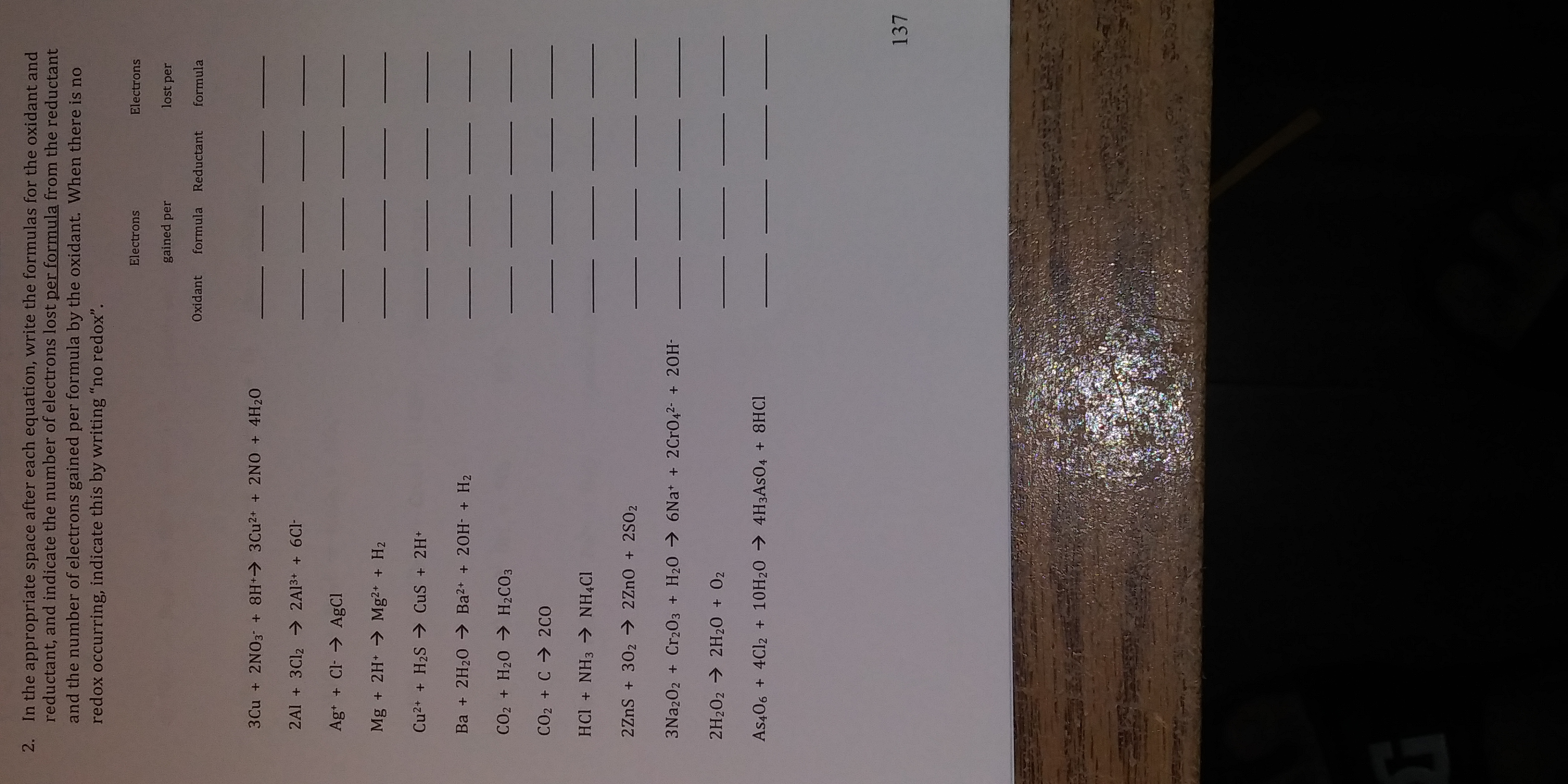# 2. In the appropriate space after each equation, write the formulas for the oxidant and reductant, and indicate the number of electrons lost per formula from the reductant and the number of electrons gained per formula by the oxidant. When there is no redox occurring, indicate this by writing "no redox" Electrons Electrons gained per lost per Oxidant formula Reductant formula Ag + Cl AgCl Mg + 2H Mg2 H2 Ba + 2H20 Ba2 20H + H2 CO2 + H20 → H2CO3 CO2 C2CO 2H202 2H20+ 02 137

Question

Number 2help_outlineImage Transcriptionclose2. In the appropriate space after each equation, write the formulas for the oxidant and reductant, and indicate the number of electrons lost per formula from the reductant and the number of electrons gained per formula by the oxidant. When there is no redox occurring, indicate this by writing "no redox" Electrons Electrons gained per lost per Oxidant formula Reductant formula Ag + Cl AgCl Mg + 2H Mg2 H2 Ba + 2H20 Ba2 20H + H2 CO2 + H20 → H2CO3 CO2 C2CO 2H202 2H20+ 02 137 fullscreen

### Want to see the step-by-step answer?